Line Studies Previous  Next

Line Studies
Line studies are lines and various geometrical shapes that can be imposed into a price or indicator chart. They include support/resistance lines, trend lines, as well as Fibonacci's, Gann's, and Andrews' tools, etc.
Line studies are collected in the "Tools" toolbar on the left of the program. To impose an object, one has to press the corresponding toolbar button (the buttons are given in the leftmost column of the table).

 Menu Command Description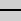Horizontal Line Horizontal line can be used to mark various levels, particularly, those of support/resistance. One point must be set for this object to be imposed.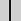Vertical Line Vertical line can be used to mark various borders in the time axis and to compare signals of indicators to price changes. One point must be set for this object to be imposed.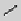Trendline Trendline helps to explore trends in price changes. Two points must be set through which a trendline will be drawn.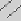Equidistant Channel Lines of the equidistant channel are always parallel. Two points must be set for this tool to be drawn.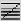Fibonacci Retracement Leonardo Fibonacci is considered to have discovered a number sequence where each successive number represents a sum of two preceding ones: 1, 1, 2, 3, 5, 8, 13, 21, 34, 55, 89, 144, etc. Each number is approximately 1.618 times more than the preceding one, and each number makes approximately 0.618 of the successive one. The tool can be drawn on two points that determine the trendline. At that, horizontal lines that meet the trendline at Fibonacci levels (retracement) as 0.0%, 23.6%, 38.2%, 50%, 61.8%, 100%, 161.8%, 261.8%, and 423.6% are drawn automatically.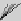Fibonacci Fan Fibonacci Fan is drawn on two points that define the trendline. Then an «invisible» vertical line is drawn through the second point. Then three trendlines are drawn from the first point, these trendlines meeting the invisible vertical line at Fibonacci levels of 38.2%, 50%, and 61.8%. It is considered that significant price changes should be expected near these lines.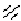Andrews' Pitchfork This tool is drawn on three points and represents the parallel trendlines. The first trendline starts at the selected leftmost point (it is an important peak or trough) and is drawn precisely between two rightmost points. This line is the pitchfork «helve». Then, the second and the third trendlines outgoing from the above-mentioned rightmost points (significant peak and trough) are drawn in parallel to the first trendline. These lines are the pitchfork «teeth». Andrews' Pitchfork is interpreted as support/resistance lines are normally interpreted.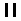Time cycle Lines This tool represents a row of vertical lines placed at equal intervals. Normally, a unit interval corresponds with one cycle. At that, completed lines are considered to describe future cycles. The tool is drawn on two points that define the unit interval.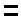Price cycle Lines This tool represents a row of horizontal lines placed at equal intervals. Normally, a unit interval corresponds with one cycle. The tool is drawn on two points that define the unit interval.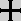Aiming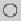Circle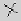Cross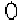Ellipse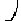Exponential curve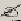Hand draw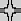Inverse circle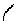Logarithmic curve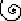Logarithmic spiral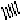Oriented cycles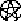Pentagram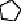Polygone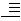Price extensions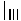Time extensions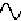Sinusoid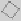Square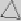Equilateral triangle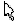Circle Draw a circle from center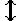Cross Draw a cross with ajustable settings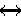Inverse circle T TextSelection Used to show a bar info or to copy a trendline with a right click Color Color F FontAiming Show time and price info for both canvas with a click on the mouse wheel Clr Clear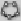Hand draw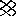SinusoidLogarithm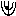Exponent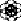Price cycles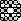Reverse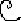Flip SQ9F Square of 9 Floating SQ144F Square of 144 Floating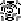Time cycles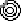Square from center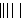Triangle from center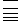Conic from 5 points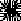Gann grid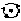Speed resistance lines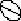Fibonacci arcs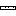Seed of life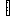Gann squareFibonacci spiralArchimed spiralFibonacci Circles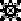Fibonacci Time Zones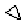Fibonacci Price Zones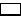Prime numbers spirals toolbox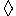Circle given 3 points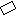Rotated ellipses VT Vector Text Vector Text: The background color is transparent. You can stretch and rotate the text.